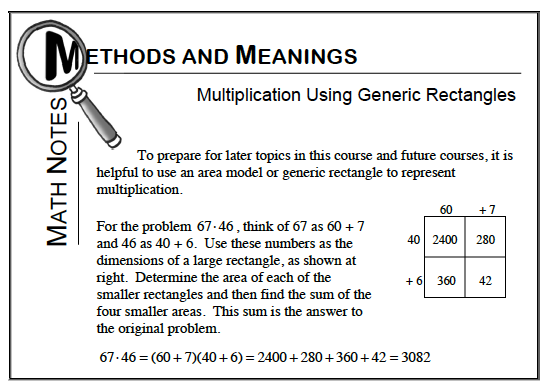### Home > CC1 > Chapter 6 > Lesson 6.1.3 > Problem6-42

6-42.

Draw generic rectangles to calculate each of the following products. What is each product? Homework Help ✎

1. $11 \cdot 33$

Refer to the Math Notes box below for help with generic rectangles.

11(33) = 300 + 30 + 30 +3 = 363

1. $111 \cdot 333$

See part (a).